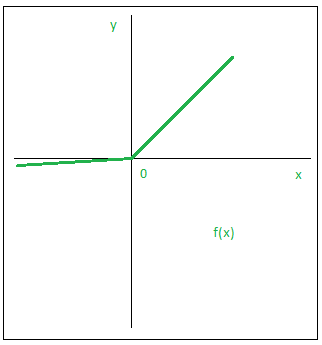Open In App
Related Articles
• Write an Interview Experience
• Deep Learning Tutorial

# Activation Functions

To put in simple terms, an artificial neuron calculates the ‘weighted sum’ of its inputs and adds a bias, as shown in the figure below by the net input.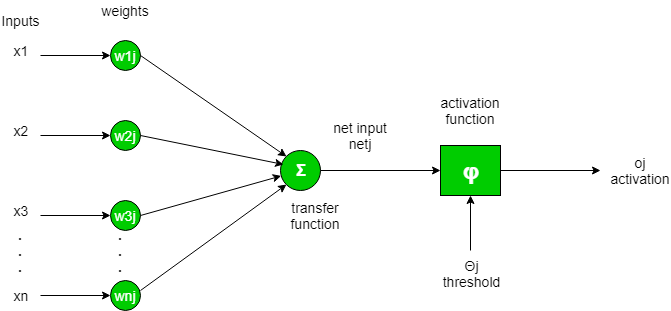Mathematically,Now the value of net input can be any anything from -inf to +inf. The neuron doesn’t really know how to bound to value and thus is not able to decide the firing pattern. Thus the activation function is an important part of an artificial neural network. They basically decide whether a neuron should be activated or not. Thus it bounds the value of the net input.
The activation function is a non-linear transformation that we do over the input before sending it to the next layer of neurons or finalizing it as output.

Types of Activation Functions –
Several different types of activation functions are used in Deep Learning. Some of them are explained below:

1. Step Function:
Step Function is one of the simplest kind of activation functions. In this, we consider a threshold value and if the value of net input say y is greater than the threshold then the neuron is activated.

Mathematically,Given below is the graphical representation of step function.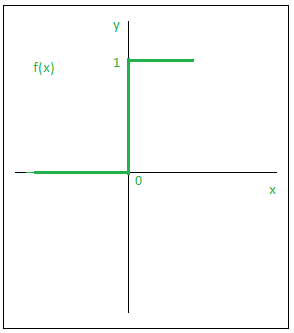2. Sigmoid Function:
Sigmoid function is a widely used activation function. It is defined as:Graphically,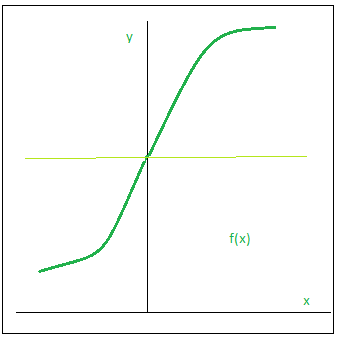This is a smooth function and is continuously differentiable. The biggest advantage that it has over step and linear function is that it is non-linear. This is an incredibly cool feature of the sigmoid function. This essentially means that when I have multiple neurons having sigmoid function as their activation function – the output is non linear as well. The function ranges from 0-1 having an S shape.

3. ReLU:
The ReLU function is the Rectified linear unit. It is the most widely used activation function. It is defined as:Graphically,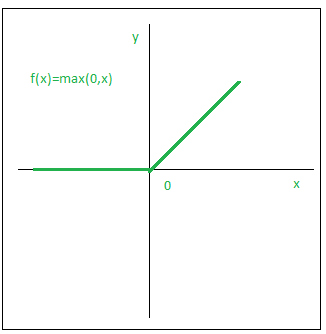The main advantage of using the ReLU function over other activation functions is that it does not activate all the neurons at the same time. What does this mean ? If you look at the ReLU function if the input is negative it will convert it to zero and the neuron does not get activated.

4. Leaky ReLU:
Leaky ReLU function is nothing but an improved version of the ReLU function.Instead of defining the Relu function as 0 for x less than 0, we define it as a small linear component of x. It can be defined as:Graphically,